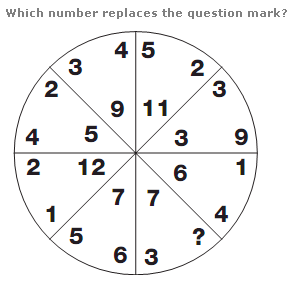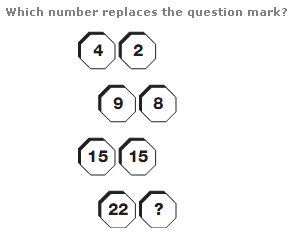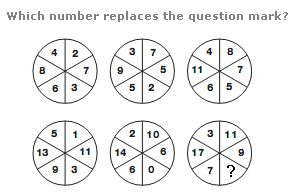# Puzzles - Number puzzles

### Exercise :: Number puzzlesAnswer : 6 Explanation : The number at the centre of each segment equals the sum of the numbers on the outside of the opposite segment.Answer : 23 Explanation : Looking at the diagram as two columns, numbers in the left hand column increase by 5, 6, 7 etc, and numbers in the right hand column increase by 6, 7, 8 etc.Answer : 5 Explanation : The numbers in the lower circles equal the numbers in corresponding segments in the upper circles multiplied by two, then subtract 3 for the left hand circle, 4 for the middle and 5 for the right hand circle.